# A Simplified Synthesis of Financial Options Pricing

[Total: 29    Average: 3.3/5]

Financial options (the right to purchase (“call“) or sell (“put“) stock (or other assets)) at a fixed price at a future date have been around for a long time. They are attractive for the option buyer since his/her total risk is the premium (price) paid for the option, as contrasted with futures contracts which are obligations to buy a stock or commodity at a future date.  However, until recently, financial options  pricing presented great difficulties and controversy: how do you put a fair price on an asset deliverable at a future date, sometimes years ahead?  Then in 1973 Drs. F. Black, M. Scholes and  R.C. Merton published a model for options pricing based essentially on Gaussian (random-walk) probability.

The following is the famed Black-Scholes equation for determining the “fair” price of a call or put option.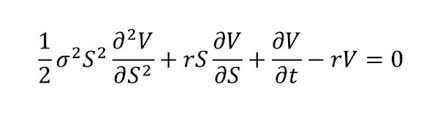Some of the  involved math may be gleaned from the following excerpt of its derivation: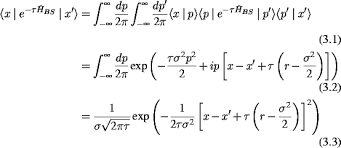And finally the formula itself: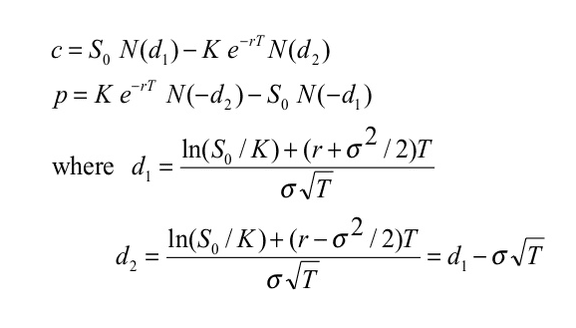where c is the cost of a call option and p the cost of a put option. I  won’t discuss the remaining parameters since we won’t be using this model directly.

As a purely theoretical inquiry, what mathematics lie behind the Black-Scholes formula?  I have long wondered and marveled at its complexity.  After all, it’s supposedly based on Gaussian probability aka random walk. Certainly the formula does not lend itself to a ready understanding of what lies behind rational options pricing.  Is there a simpler, more intuitive approach yielding the same quantitative results coupled with deeper insight into options pricing rationale?  I propose one herewith.

I am assuming a very basic familiarity with put and call options  All you need to know is spot price, strike price, duration and (annualized) volatility.   By “volatility” the financier specifically means the standard deviation of the spread of underlying  stock prices over a 1 year period.    (Interest rate is usually another input and will be touched on later.)

At this point I need to mention that the sole option price that has to be determined is the call price.  The put price for the same input parameters is immediately derivable from the so-called put-call parity rule, which is just (call price) – (put price) = (spot price) – (strike price).  So I will limit myself to call options.

Another thing to mention is that the below applies strictly speaking to so-called European options only; however, there is very little difference in price between these and so-called American options, usually well below 1%.  An online Black-Scholes options price calculator makes this clear.  Another easy-to-use Black-Scholes calculator for call options is at mystockoptions.com.

Let us go by way of an example.  Assume the spot price is $20 and the strike price$18; also that the volatility is $1 or 1/20 = 5% on a one-month basis (which is ##\frac 1 {\sqrt12}##of the annualized volatility). This means the mean is$20 and the standard deviation for 1 month of data is $1. See fig. 1.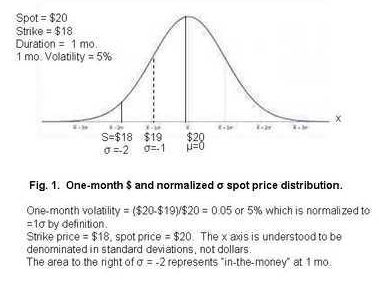We will now develop the necessary data to determine the price of a 3 month call option. At three months the strike price will represent less than 2 std. deviations since the price structure will now be “spread out” by a factor of ##\sqrt 3##. The 3 month distribution will then look like fig.2.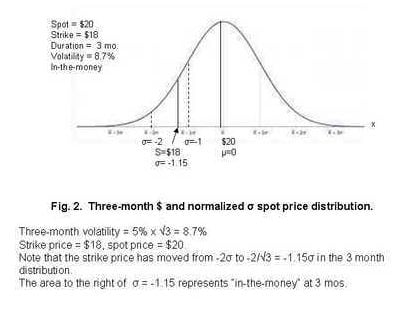Notably, the$18 strike price now represents not -2σ but -2/##\sqrt 3## = -1.15σ.  So the area under the probability density function to the right of that value represents the probability that the price will be > strike price after 3 months in which case the option has value.

But that is not the whole story.  The other question is “ok, but what will be the average value assuming the option does have value?  Clearly we want the average of the x axis to the right of the strike price, i.e. x adjusted such that at x = -1.15 the contract value is 0.

So let’s do some probability.  Let f(x) be the probability density function and F(x) the cumulative function, i.e. the area from -##\infty## to x.  The entire area = 1 so ##F(\infty)## = 1.  Then the average value of x such that F(-1.15) = 0 will be, noting that F(-x) = F(1-x),

$$\bar x = \int_{-1.15}^{\infty} (x – (-1.15)) f(x) \, dx$$

$$= 1.15\int_{-1.15}^{\infty} f(x) \, dx + \int_{-1.15}^{ \infty} x f(x) \, dx.$$

So what to do with the last integral?  No tables, nothing!  But we are saved by a very felicitous relation which is x f(x) = – f ‘(x)

so now we get

$$\bar x = 1.15\int_{-1.15}^{\infty} f(x) \, dx \text{} ~ – \int_{-1.15}^{\infty} f ‘(x) \, dx.$$    or

$$\bar x = 1.15F(1.15) – [(f(\infty) – f(1.15)]$$

= 1.15F(1.15) + f(1.15)

##\bar x ## = 0.875 + 0.206 = 1.212

Finally we multiply by the dollar value corresponding to ##\sqrt 3 ## to get cost c = ($1.73)(1.212) =$2.09.

Comparing this with the Black-Scholes calculator with the substitution ## \sigma_{1 yr} = \sqrt{(12/3)}\sigma_{3 mos.} ## to  annualize the volatility we get $2.09. Exactly the same price as ours. Let’s proceed with an out-of-the-money case, i.e. when the strike price is above the spot price. For this I chose as follows: spot =$20

strike = $25 1 month volatility =$2 = 1σ

duration = 3 mos.  See fig. 3.  This is very much an out-of-the-money option, obviously.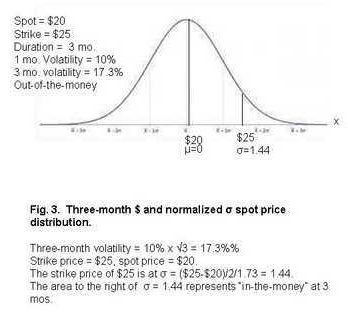The approach is the same:  the integration to obtain average x again starts at the σ equivalent of the strike price which will be ($25-$20)/2.5/1.73 = 1.44.  So to find the average x starting at 1.44 we need

$$\int_{1.44}^{\infty} (x – 1.44) f(x) \, dx$$ = f(1.44) – 1.44(1 – F(1.44)) = .142 – 1.44(0.075) = 0.034 x ($2 x 1.73) =$0.11 vs. $0.19 for the Black-Scholes model. Presumably, roundoff error accounts for the difference. In general the price of any call option will be $$c = \left( \frac \sigma \right) \int_{\sigma_{strike}}^{\infty} (x – \sigma_{strike}) f(x) \, dx$$ with ## \sigma = \sqrt{n/\sigma_v} ## , n the duration in years and ## \sigma_v ## = (annualized) volatility. I further tested the model with spot = strike =$20, and got $0.69 which agreed exactly with the Black-Scholes calculation. The prevailing interest rate should figure in these computations. It’s easily taken care of by simply accounting for “present value” of the payout. The same can be said for inflation, which at this date (Aug. 2018) is actually greater than the “safe” short-term interest rate. I’ve appended an excel file ## ^{(1)} ## that enables these computations for any combination of spot, strike, duration and volatility. (The program delineates between in-the-money and out-of-the-money cases). Should this model be used for options pricing rather than the Black-Scholes? No. The latter has been assiduously nurtured over the decades and can allegedly handle more input parameters. Online calculators facilitate its application. The point of this investigation is rather to gain insight into options pricing mathematics by formulating a much simplified basis of evaluating options, based on Gaussian variation like the Black-Scholes model, yet yielding the same results as the latter model in a much more understandable way. Then too, how valuable is any model based on random theory really? The infamous collapse of the hedge fund Long-Term Capital Management in 1999, in which Dr. Scholes was a participating investor, answers that question nicely, as does the 2008 general financial collapse. I recommend reading N. Taleb’s The Black Swan for casting further doubt on the predictability of any stochastic future pricing models. He shows that there can be and usually are severe bumps on those cherished smooth Gaussian curves! Resources: Additional (duplicate) ppt slides for the three embedded figures. These are of better quality than the embodied ones: blk-sch.ppt 19 replies 1.andrewkirk says: I like the approach of providing intuition by describing how the model works for a very short-term option, so that discounting can be ignored. That makes it a lot easier to grasp. The para that deals with interest rates (it starts with 'The prevailing interest rate should figure in these computations') is not quite right. The interest rate is the most complex part of the calculation. It is where the concept of changing the measure using the Cameron-Martin-Girsanov theorem comes in. It involves advanced concepts like equivalent martingale measures. So I would not say it is easily taken care of. The way to choose the interest rate is quite nuanced, and it affects not only the discounting of cash flows but also the assumed growth rate for the underlying stock, which determines how the mean of the bell curve shifts rightwards based on the time to option expiry. In the short-term example the bell curve does not shift at all, because the term to expiry is short enough to ignore expected growth. You could deal with that by writing a para something like: 'In the above examples we have ignored interest rates and expected growth of the stock, because they will not be significant over a short period. For most options these need to be taken into account, and that is where the additional complexity comes in. But the principle is the same as in the above examples: measuring the expected payoff over a bell curve based on the final stock price.' •rude man says: The Black-Scholes model also ignores expected growth, at least the on-line calculators do. I suppose that is one reason why the full Blk-Sch model is so much more complex. Barring growth I see nothing wrong with using present value to take care of interest. Also, no one else seems to take inflation into account which nowadays is a much greater cost determinant than the short-term, safe interest rate. The other thing I omitted was of course dividends. This is a real problem since ex-dividend stock prices typically plunge. I don’t know if Black-Scholes deals with that. Anyway, as I tried to emphasize, I was not trying to out-gun Blk.-Sch., just trying to help people see the big picture. Thanks for your expert comment! 2.rude man says: EDIT occured shortly after publication (2018-08-18 19:15 GMT). 3.alan2 says: But of course, we know that option pricing doesn't actually work. Just like MPT and CAPM it is fun math but it doesn't reflect reality. •rude man says: Absolutely correct! Couldn’t agree more. It’s fun math, is all. But it is fun! 4.andrewkirk says: rude man The Black-Scholes model also ignores expected growth, at least the on-line calculators do. It doesn't ignore expected growth. It assumes that the stock will grow at a rate equal to the risk-free interest rate minus the dividend rate (or other net benefits of holding the assets). The reasoning behind that assumption is the main insight that the original Black-Scholes paper introduced. Discussing it is beyond the scope and purpose of your note, but I think it is important to acknowledge that growth is not ignored but rather assumed to be at that rate. The expected growth cancels out against the discounting of the payout on maturity, leaving a discount factor on the first term in the formula that is ##e^{-uT}## where ##T## is time to option maturity and ##u## is the dividend rate. In examples where the underlying asset has negligible dividends or storage costs (eg some tech stocks, and commodities like gold with low ratios of storage cost to value) that discount factor is approximately 1 and is omitted. That's why it can look like growth is being ignored even though it isn't. Inflation doesn't need to be explicitly accounted for in pricing options because it is automatically incorporated into the interest rate and hence also the expected growth rate. alan2 But of course, we know that option pricing doesn't actually work. Just like MPT and CAPM it is fun math but it doesn't reflect reality. It depends what is meant by 'work'. Banks around the world use option pricing techniques like Black-Scholes to set prices for the hedging deals they offer their business customers, and they make consistent big profits with it. Usually it's a good predictor. But like all models, there are rare circumstances where it breaks down, and that's where one can lose big money. Long-Term Capital Management was a famous case of that. 5.rude man says: “Inflation doesn’t need to be explicitly accounted for in pricing options because it is automatically incorporated into the interest rate and hence also the expected growth rate.” Not so. As I said, current safe short-term interest rates are far below inflation. The former about 1.5%, the latter close to 4%. 6.andrewkirk says: rude man current safe short-term interest rates are far below inflation. The former about 1.5%, the latter close to 4%. I don't know which economy you're referring to, or which inflation measure, but all the inflation measures I know of* are about what has happened in the recent past, whereas interest rates relate to what is expected in the future. So the two are not comparable. The Black-Scholes option pricing formula is based on the construction of an arbitrage with a dynamic hedging portfolio that replicates the performance of the option. Inflation doesn't need to be considered because, regardless of what inflation does, the hedging portfolio will replicate the value of the option, and the value of a particular hedging portfolio can be explicitly observed based only on current stock prices and interest rates. * Other than the expected-inflation component of the yield on an inflation-linked bond. But those bonds are unusual and I doubt that is what the 4% relates to. •rude man says: I think we’re drifting far afield. I’ve enjoyed the conversation. 7.rude man says: andrewkirk rude man current safe short-term interest rates are far below inflation. The former about 1.5%, the latter close to 4%. I don't know which economy you're referring to, or which inflation measure, but all the inflation measures I know of* are about what has happened in the recent past, whereas interest rates relate to what is expected in the future. So the two are not comparable. The Black-Scholes option pricing formula is based on the construction of an arbitrage with a dynamic hedging portfolio that replicates the performance of the option. Inflation doesn't need to be considered because, regardless of what inflation does, the hedging portfolio will replicate the value of the option, and the value of a particular hedging portfolio can be explicitly observed based only on current stock prices and interest rates. * Other than the expected-inflation component of the yield on an inflation-linked bond. But those bonds are unusual and I doubt that is what the 4% relates to. "I don't know which economy you're referring to, or which inflation measure, but all the inflation measures I know of* are about what has happened in the recent past, whereas interest rates relate to what is expected in the future. So the two are not comparable." ??? They don't change that fast! If I pay x$ at t=0 and cash in at t=1 year, the inflation will very probably be very much at t=1yr as at t=0.

8.andrewkirk says:
rude man

First you say inflation is incorporated into interest rates, then you they're not comparable.

No, I didn't say the second. I said that past inflation is not comparable with future interest rates. There is a relationship between inflation expectations and interest rates but we need to compare them for the same period.

9.DrDu says:

When I first heard about the Black Scholes theory, it was formulated in therms of stochastic or Ito-integrals, which are quite unfamiliar to physicists. The excerpt from the derivation which you show seems to use some bracket formalism a la QM. Could you provide a source?

10.bhobba says:

Nice – its something everyone should know about – but only a few ever will – which is a pity.

alan2

But of course, we know that option pricing doesn't actually work. Just like MPT and CAPM it is fun math but it doesn't reflect reality.

Yes – as was aluded to in the article and related to it being 'wrong' due to the phenomena of fat tails – which is deeply rooted in the central limit theorem that you need to really understand to know why the beautiful math is actually a chimera. Mandelbrot and others warned about it:
https://users.math.yale.edu/mandelbrot/web_pdfs/mildvswild.pdf

Again, as alluded to in the article, it Is also is heavily implicated in the 2008 market crash:
http://web.mit.edu/wangj/www/pap/HW_070228.pdf

I am unsure to give as the reason why people should know about it as its foolish application despite warnings from very distinguished mathematicians like Mandelbrot, or the actual beautiful, but flawed math itself.

Thanks
Bill